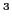Next: Continuity equation in the Up: Continuity equation and local Previous: Continuity equation and local

# introduction

In recent years, methods using energy density functionals (EDFs)  to describe nuclear properties are being developed in three complementary directions. First, the ideas of effective theories [G.P. Lepage, Lectures given at the VIII Jorge Andre Swieca Summer School (Brazil, 1997), nucl-th/9706029(),2] are employed in determining the EDFs from first principles [3,4,5,Stoitsov et al.(2010)Stoitsov, Kortelainen, Bogner, Duguet, Furnstahl, Gebremariam, and Schunck]. These developments are supplemented by a renewed interest [Dobaczewski et al.(2010)Dobaczewski, Carlsson, and Kortelainen,Carlsson and Dobaczewski(2010),Gebremariam et al.(2010)Gebremariam, Duguet, and Bogner] in the density-matrix expansion (DME) methods [Negele and Vautherin(1972),Negele and Vautherin(1975)], which allow for treating exchange correlations in terms of (quasi)local functionals. Second, the coupling constants of the well-known EDFs undergo a thorough scrutiny, including an advanced work on the readjustment of parameters [Erler et al.(2010)Erler, Klüpfel, and Reinhard,6] and study of inter-parameter correlations . Finally, the standard functionals are extended by adding new terms [Carlsson et al.(2008)Carlsson, Dobaczewski, and Kortelainen,9,8,Raimondi et al.(2011)Raimondi, Carlsson, and Dobaczewski], so as to gain increased precision of description and predictability, in quest for the spectroscopic-quality  and universal [Bertsch et al.(2007)Bertsch, Dean, and Nazarewicz] EDFs.

In the present work we study properties of EDFs [Carlsson et al.(2008)Carlsson, Dobaczewski, and Kortelainen] and pseudopotentials [Raimondi et al.(2011)Raimondi, Carlsson, and Dobaczewski] extended by adding terms that depend on higher-order derivatives up to sixth, next-to-next-to-next-to-leading order (NLO). Such extensions lead to self-consistent mean-field Hamiltonians that are sixth-order differential operators , that is, they depend on up to sixth power of the momentum operator. This makes them unusual objects, in the sense that standard second-order one-body Hamiltonians contain only the Laplace operator in the kinetic-energy term and possibly the angular-momentum operator in the spin-orbit term. The main question we address here is whether the presence of higher powers of momenta is compatible with the continuity equation (CE).

The CE is a differential equation that describes a conservative transport of some physical quantity [K. F. Riley, M. P. Hobson and S. J. Bence,Mathematical Methods For Physics And Engineering, (Cambridge Univ. Press, Cambridge, 2008)()]. In quantum mechanics, it relates the time variation of the probability density to the probability current . In our case, it appears when the NLO EDFs or pseudopotentials are employed within a time-dependent theory. For the standard Skyrme (NLO) functional, the validity of the CE has been checked explicitly [Engel et al.(1975)Engel, Brink, Goeke, Krieger, and Vautherin]. Our goal here is to derive constraints on the coupling constant of the NLO EDF or parameters of the pseudopotential that would guarantee the validity of the CE. Apart from linking the CE to the local gauge symmetry , we also analyze the CEs in vector and isovector channels and link them to the local non-abelian gauge symmetries.

The paper is organized as follows. In Sec. 2.1 we present the standard quantal CE for a single particle and introduce the vector CE. Then, in Sec. 2.2 we discuss the CEs within the time-dependent density functional theory and in Sec. 2.3 we specify the case to the NLO quasilocal functional. The main body of results obtained for the CEs in the four spin-isospin channels is presented in Sec. 3 and Appendices A-C. Finally in Sec. 4 we formulate the conclusions of the present study.Next: Continuity equation in the Up: Continuity equation and local Previous: Continuity equation and local
Jacek Dobaczewski 2011-11-11